Impact Factor 2.073
2017 JCR, Clarivate Analytics 2018

The world's most-cited Neurosciences journals

Frontiers in Computational Neuroscience

Original Research ARTICLE

Front. Comput. Neurosci., 10 November 2011 | https://doi.org/10.3389/fncom.2011.00047

Synaptic scaling in combination with many generic plasticity mechanisms stabilizes circuit connectivity

• 1 Institute for Physics – Biophysics, Georg-August-University, Göttingen, Germany
• 2 Network Dynamics Group, Max Planck Institute for Dynamics and Self-Organization, Göttingen, Germany
• 3 Bernstein Center for Computational Neuroscience, Georg-August-University, Göttingen, Germany
• 4 Institute for Physics – Non-linear Dynamics, Georg-August-University, Göttingen, Germany

Synaptic scaling is a slow process that modifies synapses, keeping the firing rate of neural circuits in specific regimes. Together with other processes, such as conventional synaptic plasticity in the form of long term depression and potentiation, synaptic scaling changes the synaptic patterns in a network, ensuring diverse, functionally relevant, stable, and input-dependent connectivity. How synaptic patterns are generated and stabilized, however, is largely unknown. Here we formally describe and analyze synaptic scaling based on results from experimental studies and demonstrate that the combination of different conventional plasticity mechanisms and synaptic scaling provides a powerful general framework for regulating network connectivity. In addition, we design several simple models that reproduce experimentally observed synaptic distributions as well as the observed synaptic modifications during sustained activity changes. These models predict that the combination of plasticity with scaling generates globally stable, input-controlled synaptic patterns, also in recurrent networks. Thus, in combination with other forms of plasticity, synaptic scaling can robustly yield neuronal circuits with high synaptic diversity, which potentially enables robust dynamic storage of complex activation patterns. This mechanism is even more pronounced when considering networks with a realistic degree of inhibition. Synaptic scaling combined with plasticity could thus be the basis for learning structured behavior even in initially random networks.

1. Introduction

Neural systems regulate synaptic plasticity avoiding overly strong growth or shrinkage of the connections, thereby keeping the circuit architecture operational. Accordingly, experimental studies have shown that synaptic weights increase only in direct relation to their current value, resulting in reduced growth for stronger synapses (Bi and Poo, 1998; Sjöström et al., 2001; Frömke et al., 2005). It is, however, difficult to extract unequivocal evidence about the underlying biophysical mechanisms that control weight growth.

The theoretical neurosciences have addressed this problem by exploring mechanisms for synaptic weight change that contain limiting factors to regulate growth. Most basic limiting mechanisms act subtractively or multiplicatively (Bienenstock et al., 1982; Oja, 1982; Miller and MacKay, 1994) such that individual weight growth decreases with the total synaptic weight in a network. Other mechanisms, such as additional thresholds in input or output (Sejnowski and Tesauro, 1989; Gerstner and Kistler, 2002), yield a similar weight-limiting effect. The effectiveness of such mechanisms notwithstanding, some are difficult to justify from a biophysical perspective, in particular those that require knowledge of global network status (e.g., knowledge of the “sum of all weights”) for normalization.

The discovery of spike-timing dependent plasticity (STDP; Gerstner et al., 1996; Magee and Johnston, 1997; Markram et al., 1997) enabling weight growth as well as shrinkage, has offered a potential alternative solution to the problem because STDP can stabilize synaptic weight distributions (Song et al., 2000; Van Rossum et al., 2000; Gütig et al., 2003; Clopath et al., 2010). However, stability is not always guaranteed by STDP because various types of plasticity exist across different neurons and even at the same neuron, depending on the location of the synapses (Frömke et al., 2005; Bender and Feldman, 2006; Sjöström and Häusser, 2006). Weight dependent STDP has been introduced to solve this issue (Van Rossum et al., 2000; Gütig et al., 2003).

In 1998, a series of studies initiated by Turrigiano augmented this discussion by demonstrating that network activity is homeostatically regulated (Turrigiano et al., 1998; Turrigiano and Nelson, 2004; Stellwagen and Malenka, 2006), where overly active networks will down-scale their activity and vice versa. This results from synaptic scaling suggesting that weights w are regulated by the difference between actual and target activity (≈vvT).

Here we suggest that synaptic scaling is combined with different types of plasticity mechanisms in the same circuit or even at the same neuron and regulates synaptic diversity across the circuit. Our study demonstrates that synapses are stabilized strictly in an input-determined way thereby capturing characteristic features of the inputs to the network. As an interesting result, we show that such systems are capable of representing a given input pattern via stably changed weights along several stages of signal propagation. This holds even in circuits containing a substantial number of random recurrent connections but no particular additional architecture.

2. Materials and Methods

We will first describe the mathematical framework which leads to the analytical as well as numerical observations in the Results Section, a few important equations are repeated there, too. In the second part we will present all parameters and other constraints used.

2.1. General Derivation of Normal Form, Fixed Points, and Stability

As scaling co-acts with plasticity, such a combined mechanism is mathematically characterized in its most general additive form by a weight change: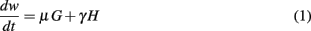where w is the synaptic weight. Here μ and γ define the rates of change of conventional synaptic plasticity and scaling, γ ≪ μ ≪ 1, and G and H represent the specific types of plasticity and scaling (Abbott and Nelson, 2000; Turrigiano and Nelson, 2000), respectively. For example, G is different for plain Hebbian plasticity (Hebb, 1949) and for STDP.

Experimental results suggest that synaptic scaling compares output activity v against a desired target activity vT of each individual neuron. Most straightforwardly, such a local weight change is defined by: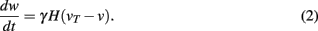The output v is written in a more general way by defining F as the neuronal activation function of the neuron, with u its input, giving us v = F(u) and: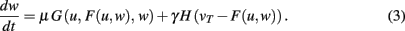This equation describes the basic, general dynamics that combines conventional plasticity G and synaptic scaling H, which we first define in a weight-dependent way, as suggested by others (Abbott and Nelson, 2000; Turrigiano and Nelson, 2004):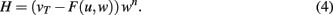We insert this into Eq. 3 and obtain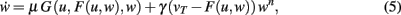where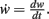The output dynamics at typical neuronal operating points are naturally proportional to the synaptic strength for given inputs; we thus take F(u,w) to be arbitrarily non-linear in the input but proportional to the synaptic weight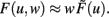Therefore, Eq. 5 simplifies to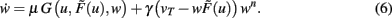One goal of the current study is to show that synaptic scaling can stabilize a wide variety of learning rules. Thus, in the next step we consider basic plasticity rules, hence, rules that are initially unstable as they do not contain additional stabilization terms. These are for example plain Hebbian and Anti-Hebbian learning, BCM without sliding threshold, plain spike-timing dependent plasticity, and others. All these rules strictly obey a second order polynomial of the weight of the following kind.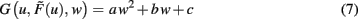where a, b, and c are functions of the activities u and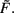The w-independent term is taken to be c = 0, because biophysically the weight of a non-existing synapse naturally cannot grow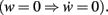Thus,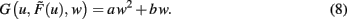This second order equation (Eq. 8) acts as the normal form for many learning rules G and captures not only Hebbian but also BCM (constant-threshold!) plasticity (see section Introducing the Framework and Appendix) as well as all other possible learning rules that have at most a second order weight dependence. Higher order dependencies occur with rules which contain stabilization terms. As we will show that synaptic scaling generically performs stabilization, additional terms are not required and we can restrict ourselves to second order dependencies here.

Inserting Eq. 8 into Eq. 6 we obtain the final form of the combined scaling and plasticity mechanisms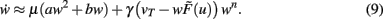This implies mathematically that weights could become negative and, therefore, the output activity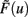can be negative, too, which is, however, just a technicality.

2.2. General Fixed Point Analysis

Fixed points of Eq. 9 are analyzed for weight-independent synaptic scaling (n = 0) as well as for linear (n = 1) and non-linear (n > 1) weight dependency. This analysis is based on standard methods for a given set of differential equations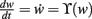determining the dynamics of w and its fixed points w*, where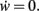To assess the stability of these fixed points, we analytically computed the Jacobian Jϒ(w) of ϒ; a fixed point w* is stable if all eigenvalues at w = w* are smaller than zero, and unstable otherwise.

Specific instantiations of synaptic plasticity mechanisms, parameters, and time axes

As neuron model most often we consider generic rate processing F = uw, but spiking neuron models yield similar results (see Figures 2C–F).

The Hebbian mechanism is defined by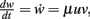with neuronal output v = F(u, w).

The constant-threshold Bienenstock-Cooper-Munro [BCM, Bienenstock et al. (1982)] rule is given by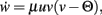where Θ is a constant-threshold. This rule shows comparable dynamics as spike-timing dependent plasticity [STDP; Markram et al. (1997); Bi and Poo (1998)] as discussed in the Discussion. Thus, we will many times treat BCM and STDP in a similar way in this paper.

The synaptic scaling mechanism H uses two parameters: γ, the scaling rate, and vT, the target firing rate of the neuron, which can be different for every neuron [e.g., Izhikevich (2004)]. Additionally, other mechanisms [e.g., intrinsic plasticity; Triesch (2007)] could dynamically adapt vT over time. However, in our numerical experiments we keep vT constant over the whole network. Assessing the influence of a dynamical vT could be part of future work. However, the reader should note that the activity of a neuron never reaches the target firing rate as long as synaptic plasticity influences the synapses (see Table 1 and Appendix).

TABLE 1

All constants (μ, γ, vT) are kept unit-free, and the resulting synaptic dynamics (Eq. 11) only depends on the ratio of time scales μ/γ. In general, we use a smaller ratio as found in experiments (see below) to speed up simulations; this mainly shortens the relaxation times toward fixed points but does not qualitatively change the phase space structure, in particular the existence and stability of fixed points.

The time scale of synaptic dynamics μ and γ is difficult to determine as different experiments show different time scales [e.g., for LTP the synaptic plasticity rate 1/μ ranges from min to h (Bliss and Lomo, 1973; Dudek and Bear, 1993) and for synaptic scaling from h to days (Turrigiano et al., 1998; Turrigiano and Nelson, 2004)]. Thus, time axes in all plots (cf. Figures 2 and 4) are scaled by simulation units.

With these definitions it is possible to analytically calculate fixed points for one or two synapses for most basic learning rules. Results are shown in Table 1 and the calculations are in the Appendix.

3. Results

We will first present some general mathematical results concerning the stability of combined plasticity and scaling mechanisms. This is supplemented by numerical experiments showing how simple single- or multi-synapse systems behave. In the next section we will reproduce some biological findings and finally we will show some predictions that arise from using plasticity and scaling in recurrent networks.

3.1. Introducing the Framework

How do combined plasticity and scaling mechanisms affect synaptic weight changes? To understand this, we analyze the dynamics of weight changes dw/dt in dependence of neuronal inputs and current synaptic weights.

In its most general additive form (see Method Section), plasticity and scaling mechanisms change synaptic weights according to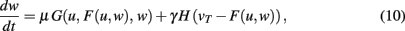where u is the input to a neuron, the function F characterizes its output given an arbitrary neural dynamics v = F(u,w), the function G specifies any generic plasticity mechanism, and H mediates synaptic scaling determined by its target firing rate (vT > 0) as introduced above.

Turrigiano and Nelson discuss that synaptic scaling should be dependent on the actual synaptic weight [see Figure 4 in Turrigiano and Nelson (2004), see also Abbott and Nelson (2000)]. It remains unknown, however, what form this dependence takes. We therefore analyze three classes of synaptic scaling mechanisms: weight-independent scaling, linear weight dependence, and non-linear weight dependence of the scaling. For the dynamics of weight changes, such scaling is expressed via a weight dependence wn, where n = 0 represents weight independence, n = 1 the linear, and n > 1 the higher order, non-linear dependence. Furthermore, experimental work by Turrigiano and Nelson (2004) suggests that the output rates of the neurons in the circuit tend toward some target rate vT. This results in the specific form of H = [vTF(u,w)]wn. Inserting H into Eq. 10, we obtain (see Materials and Methods Section):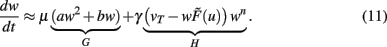where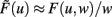and parameters a and b define the specific type of the plasticity mechanism G. For example, they will be different for plain Hebbian plasticity (a = 0;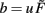) and for BCM (a ≠ 0, see below).

Synaptic scaling generically stabilizes synapses

The combined synaptic dynamics (Eq. 11) exhibit certain overarching convergence properties reflected in the structure of the phase space, as can be seen by the existence and stability of fixed points. Stable fixed points define synaptic values toward which the weights converge over time. As a central observation we find that whereas weight-independent synaptic scaling does not stabilize synapses, a convex, non-linear weight dependence generically does stabilize them. In this case stable fixed points are generated regardless of the underlying specific plasticity mechanism G and independent of the neuronal dynamics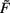(see Materials and Methods Section for a detailed derivation).

These specific results are presented in Table 1. One can see that for n = 0 and n = 1 one unstable and one stable fixed point exist.

For n = 2 existence and stability of fixed points depend on the parameters given that the firing rateand the time scales γ and μ are positive.

For b > 0 we find that Γ2 > μa + γvT and thus w*,1 is unstable, whereas w*,2 and w*,3 are stable, independent of the sign of a. For plain Hebbian plasticity, we indeed have b > 0.

For b < 0, we find that w*,1 is stable. The stability of the two other fixed point now depends on a. For a > − (γ/μ)vT, w*,2 is stable and w*,3 unstable and vice versa for a < − (γ/μ)vT. For the BCM rule we indeed have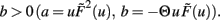The central result of this analysis is that, for n = 2, independent of the parameters and the actual plasticity rule, two fixed points are stable and one fixed point is unstable, leading to global stability, which is not the case for n < 2. In other words: synaptic scaling will globally stabilize all second order plasticity rules when scaling has a second order weight dependence (w2). This covers a very large class of experimentally observed plasticity rules such as plain Hebbian learning, BCM without sliding threshold, plain spike-timing dependent plasticity, and others.

Stabilization of systems with one or two synapses

The way synaptic scaling co-acts with synaptic plasticity relies on whether and how synaptic scaling is weight-dependent. For Hebbian plasticity, G = uv, Figure 1 illustrates a typical example for the simplest neuronal activation function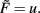Already single synapses (Figures 1A–C) follow the basic principles underlying the combined dynamics of conventional plasticity and synaptic scaling.

FIGURE 1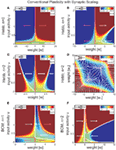Figure 1. Combined conventional plasticity and weight-dependent scaling yields stable synapses. Phase space diagrams for synaptic scaling wn with different weight-dependencies (n = 0: weight-independent scaling, n = 1: linear weight dependence, n = 2: non-linear weight dependence) and plasticity rule [Hebbian (A–D); BCM (E,F)]. Small insets (top-left of each panel) show the connectivity. White arrows indicate convergent weights, magenta arrows divergent weights. (A–C), (E,F) Weight changes in dependence of the input activity for a single input synapse. Colors indicate weight change dw/dt (blue: decrease, red: increase). White and magenta curves indicate stable and unstable fixed points of the weights, resp. (D) Simultaneous weight changes for two synapses showing one cross section through the (w1,w2,u1,u2)-phase space of a two-synapse system, fixing u1 = u2 = 1.0. Stable fixed points indicated by white disks, unstable fixed point at zero weights indicated by magenta disk. (A) one input, n = 0; (B,E) one input n = 1; (C,F) one input n = 0; (D) two inputs, n = 2, colors here indicate squared rate of change (dw/dt)2 = (dw1/dt)2 + (dw2/dt)2. Parameters: (A–F) Relative time scales between conventional plasticity and scaling μ/γ = 10, target activity vT = 0.3, (D) input activity u1 = u2 = 1.0, (E,F) Θ = 0.5.

For weight-independent scaling (n = 0, Figure 1A), the dynamics are dominated by just one unstable fixed point for all but very small input activities. Synaptic weights typically grow unboundedly and diverge to either large positive or large negative values. Thus, combining Hebbian plasticity with weight-independent scaling does not yield a globally stable system. The same holds for other forms of plasticity, for example the Bienenstock-Cooper-Munro mechanism [BCM, Bienenstock et al. (1982), not shown]. Taken together, synaptic changes due to conventional synaptic plasticity are generically not stabilized by weight-independent synaptic scaling.

Does weight-dependent synaptic scaling stabilize? Not necessarily. Linearly weight-dependent synaptic scaling (n = 1) combined with plasticity leads to stable synapses only under certain conditions. For Hebbian plasticity we observe stability only for excitatory synapses (Figure 1B), whereas for BCM plasticity (Figure 1E) we obtain large unstable regions for both inhibitory and excitatory synapses.

By contrast, synaptic scaling with a convex non-linear weight dependence n = 2 generically stabilizes globally for both, excitatory and inhibitory synapses. As we had shown analytically (see Table 1) for arbitrary neuronal activation functionthis holds independent of the used second order plasticity mechanisms. Furthermore, one can analytically show that this result also holds for higher order convex non-linearities (n ∈ {2, 4, 6…}) and that non-integer exponents yield instabilities for negative synapses, but these technical aspects are omitted here. Higher order exponents appear unlikely anyhow as the fixed point characteristic becomes more and more independent of the actual neuronal activation (input activity).

Any synaptic scaling with convex non-linear weight dependence, i.e., n = 2k, kN implies globally stable synaptic fixed points. We note below why such higher order non-linearities likely play no major role in biological neural system.

The weight dynamics and its derivative for even values of n (i.e., n = 2k) in general read as follows: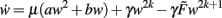The highest order term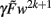(with γ > 0 and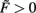) is negative for w → ∞ and positive for w → − ∞. As a result, the derivative at the largest absolute value of the roots is negative for both negative and positive values. Thus synapses do not strengthen unboundedly because |w| remains bounded.

Exponents larger than 2 are, however, likely much less essential to the description of biophysical synaptic dynamics than exponents n ≤ 2. The reasoning is as follows: Large parts of the dynamics (Figure 1C) are dominated by almost straight, tilted lines of fixed points (the exact form depends on the used neuron model; see Table 1) that occur above a certain, small neuronal activation value, e.g., u > 0.2. Similar features are also found for BCM plasticity (Figure 1F, u > 0.6). For n ≥ 4 the tilt of these lines is strongly reduced and they become more and more vertical. This results in the unrealistic situation where plasticity is almost independent of the input. Specifically, different noisy inputs will not lead to different, distinguishable weights anymore. We, thus, consider exponents substantially larger than 2 unlikely in biophysical systems.

Finally, we discuss the fixed point properties for non-integer exponents. We analyzed these systems analytically and numerically and found that for all non-integer exponents there are no real-valued fixed points in the range w < 0. For the positive range w > 0, fixed points exist and are stable for plain Hebbian plasticity and n > 0 and for BCM plasticity and n > 1. This confirms that stability is not a singular event but that it is insensitive, existing continuously within the positive weight range, even extending into the negative weight range for integer exponents.

The phenomenon of global stabilization is moreover robust to changes in time scales and works in a broad range of target activities vT as will be demonstrated by several numerical experiments below. Systems with multiple synapses exhibit qualitatively the same features of global stability, cf. Figure 1D for a two-synapse system.

Thus synaptic scaling with a convex second order weight dependence robustly stabilizes synapses, regardless of the underlying second order synaptic plasticity mechanism G (Figure 1), the type of synapse (inhibitory or excitatory), the absolute time scales of synaptic changes, the target activity of scaling, and the type of neuronal activation function (Figures 2C–F).

FIGURE 2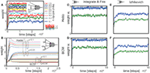Figure 2. Stabilization of synaptic weights follows the input pattern for different neuron models and are not affected by the neuron model. (A) System stabilization with one neuron that receives ten noisy inputs. Although the signal to noise distance of the input activities (shown for the two strongest inputs in the bottom inset) is very small and, therefore, the signals overlap to a large degree, the system maps them on distinguishable weights. (B) Neuron with 20 inputs, 10 following a plain Hebb rule, the other 10 the BCM rule using also different learning rates μ as indicated. (C–F) Weights stabilize also when using non-linear (spiking) activation functions F. Two neurons provide input to one target neuron. Inputs were Poisson spike trains with 2 (green) and 3 (blue) spikes per 100 simulations steps. The used neuron models are Integrate and Fire (firing threshold = 0.5) with (C) plain Hebb and (D) BCM and Izhikevich [“RS” neuron; Izhikevich (2003)] with (E) plain Hebb and (F) BCM. Parameters: Time axes in simulation steps. (A) relative time scales of plasticity and scaling μ/γ = 10, vT = 0.5, (B) μ1 = 0.1, μ2 = 0.01, γ = 0.001 resulting in a ratio of μ/γ of 100 or 10, resp., vT = 0.5, for BCM: Θ = 0.3. Each group of five inputs receives: u{1, 2, 3, 4, 5} = {0.015,0.018, 0.020, 0.022, 0.025}, (C–F) μ/γ = 10, vT = 0.1, (E,F) Θ = 0.5. For a detailed description of the used neuron models see Appendix.

Generic stabilization of multi-synapse systems

To make a network operational, many synapses need to be stabilized. It is, thus, central to understand how the effects observed above generalize to multi-synapse systems. This is non-trivial, as for two or more synapses projecting onto the same neuron, synaptic changes influence each other because all inputs determine the relation between the actual neural output v and target vT, which – in turn – regulates synaptic growth. Neural inputs u have only slow influence on the synapses, characterized by small changing rates μ and γ. Thus, we focus in the following on constant inputs and treat variations separately. An analysis similar to that for single-synapse systems (cf. Materials and Methods Section) demonstrates the generic existence of stable fixed points and numerical simulations confirm this view. For instance, Hebbian plasticity combined with non-linearly weight-dependent synaptic scaling in a multi-synapse system equally yields synapses that are both diverse and stable. This holds both for constant inputs (Figure 2B) as well as for inputs that exhibit strong variations such as noise (Figure 2A). The resulting synaptic pattern faithfully reflects the distribution of the input signals.

In general, we observe that all synapses quickly stabilize even if different plasticity mechanisms co-act and if different time scales of plasticity are involved at the same neuron (Figure 2B). Furthermore, we find that synaptic diversity stabilizes independent of the neuronal dynamics, for rate-coded neuron as well as spiking neurons (Figures 2C–F). To some degree remarkable one should note that some of these activation functions are quite non-linear, but stability is not affected.

Here, the reader should be reminded that the dynamics of the constant-threshold BCM rule are, as already mentioned in the Methods, comparable to the dynamic of STDP. This fact will be further discussed in the Discussion.

In the introduction we pointed out that neural systems are capable of stabilizing different synapse types which coexist in the network. The results shown in Figure 2 demonstrate that this is possible when combining plasticity with non-linear scaling: Synapses are stabilized at the same neuron even when different rates and different plasticity mechanisms coexist. Synaptic scaling combined with any type of plasticity thus provides a joint mechanism capable of maintaining synaptic diversity in a neural circuit.

3.2. Experimental Predictions

Existing reports that the change of a synaptic weight is inversely related to its initial size (Abraham and Bear, 1996; Bi and Poo, 1998) are confirmed by plotting dw/dt over w (Figures 3A–D, dashed curves). In this Figure, we investigate a single-synapse with Hebbian or BCM plasticity, similar to the setup in Figures 1B,C,F. If synaptic scaling is linear (n = 1), the dependency results in straight lines; for a second order characteristic (n = 2), a curved behavior is observed (compare Figures 3A,B). Curvature for a BCM rule, with n = 2 (hence, for an STDP-like behavior) is less pronounced than for a Hebb rule, though (compare Figure 3B with Figures 3C,D). The data shown in Bi and Poo (1998) had been fitted by a straight line [see Figure 5 in Bi and Poo (1998)], but the widely varying distribution of the data points would be compatible with a curved fit with small curvatures, too, such as the ones found in Figures 3C,D.

FIGURE 3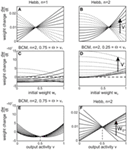Figure 3. Weight change depends on initial weight and on post-synaptic depolarization level. (A–D) Weight change dw/dt plotted against initial weight w0 for different plasticity mechanisms and different degrees of scaling. Output activity was increased as indicted by the arrows on the right. In (A,B) the horizontal line at dw/dt = 0.01 reflects no scaling as v = vT.(E,F) Replotting the data from (C,B) now showing weight change dw/dt plotted against output activity v. Initial weight was increased as indicted by the arrow on the right. Parameters: vT = 0.5, μ = 0.1, u = 0.2, (A,B,F) μ/γ = 2, (C-E) μ/γ = 10.

A pronounced LTD/LTP characteristic is observed in Figure 3C (parts of the curves are below zero), which results from a BCM rule with high threshold Θ. Such a behavior is also observed for STDP with nearly equally strong LTP and LTD parts of the learning window. Here our analysis predicts that for initially strong synapses and a high post-synaptic depolarization level, the learning window will revert. In this case, a situation which normally leads to LTP would result in LTD and vice versa (right parts of curves). Thus, synaptic scaling can lead to an inversion of the normally expected synaptic change, depending on the neuron’s output. Figure 3D shows an LTP-dominated case, where this inversion is less pronounced.

In general, for small output values v, hence low levels of post-synaptic depolarization, theoretically one should expect a different behavior resulting in weight increase even for relatively large weights (Figures 3A–D, solid curves). This will be difficult to measure experimentally though as for small depolarization levels, plasticity would be very small to begin with.

A clear differentiation between Hebbian versus BCM (or STDP) plasticity is also visible when replotting the data from Figures 3B,C now showing the weight change dw/dt plotted against v (Figures 3F,E).

The results from Figure 3 represent an ideal situation but effects similar to the ones shown here should be measurable when statistically evaluating plasticity at many synapses under different levels of depolarization of the post-synaptic neuron and with differently strong initial synaptic weights.

3.3. Synaptic Stabilization in Recurrent Circuits

In the final sections we investigate properties that arise from the combination of plasticity with scaling and which might have influence on information processing in neural structures, especially in recurrently wired networks.

The analysis of the combined scaling + plasticity rule in recurrent networks relates to the problem of how to establish cell assemblies. While it is generally acknowledged that assembly formation ought to be an important process for network function, little is known how this could be achieved in a stable and reliable way.

Here we will show that recurrent networks, where conventional plasticity mechanisms combine with scaling, are capable of mirroring their inputs at the synapses such that these systems can learn to embed an input pattern in their connectivity. This happens even in recurrent networks that are random and not specifically pre-structured.

Analyzing small circuits helps understanding the basics of these features. Figure 4 displays a reciprocally connected pair of neurons with one external input each, where either the reciprocal synapses only (Figures 4A,B) or all synapses (Figures 4C,D) may change. Depending on the input differences, strong or weak synapses are stabilized and more or less bi-directionality of this neuron pair is achieved. As pointed out earlier, such systems stabilize regardless of the precise rates of change γ, and insensitive to the values of vT. There may still occur an initial overshoot of some weights if vT is too large. Purely excitatory, especially recurrent, networks with substantially lower vT avoid such overshoots. Whether or not such overshoot may occur in biological circuits needs to be experimentally tested. Perhaps, avoiding overshoot requires unrealistically small vT. This requirement, however, immediately disappears in the presence of inhibition. As Figure 4E illustrates, the same circuit with an additional inhibitory neuron yields stable weights without overshoot at approximately the same values as the original circuit (Figure 4D). Thus, inhibition has the capability to yield stable synapses for a range of reasonable target activities and, furthermore, minimize the emergence of divergent weights. Here we have vT = 0.5, similar to the values used for purely feed-forward systems (Figures 1 and 2). This finding sheds a new light on the role of inhibition in neural circuits and suggests that inhibition may help stabilizing synaptic weights in conjunction with synaptic scaling.

FIGURE 4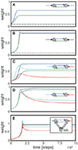Figure 4. Stabilization of bi-directional, recurrent connections. (A,B) Input synapses (dashed lines) are fixed. (A) displays synaptic dynamics for dissimilar inputs, (B) for similar inputs. (C,D) Input synapses are also allowed to change. (C) displays synaptic dynamics for dissimilar inputs, (D) for similar inputs. (E) as (D) but with one inhibitory neuron. Parameters: (A–D) relation between plasticity and scaling μ/γ = 10, vT = 0.007. (A) u{1, 2} = {0.01, 0.001} (B) u{1, 2} = {0.002, 0.001} (C) u{1, 2} = {0.01, 0.001} (D,E) u{1, 2} = {0.002, 0.001}. (E) vT = 0.05, excitatory-to-inhibitory connections are set to w3,1 = w3,2 = 0.15, and inhibitory-to-excitatory connections are w1,3 = w2,3 = 1.0, all unchanging.

3.4. Weight Predictions Agree with Experiments

The system introduced above in a recurrent network reproduces key qualitative features of synapses observed in experiments (Figure 5). The theoretical model with Hebbian plasticity and synaptic scaling with n = 2 (used parameters as for Figures 1C,D) correctly predicts both, the roughly linear increase or decrease of synaptic weights with circuit activity (Figure 5A) and the overall synaptic weight distribution (Figure 5B) found experimentally.

FIGURE 5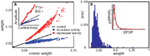Figure 5. Model predictions are consistent with experimental findings. (A) Decrease or increase of network activity proportionally changes synaptic weights. In experiment, Tetrodotoxin (TTX) inhibits network activity, whereas Bicuculline (BIC) disinhibits it. A recurrent network with random connectivity and plastic synapses behaves comparable to the experimental data. Here, each neuron receives an external input which is decreased (red) or increased (blue) compared to a control case (black line). [inset, modified from Turrigiano et al. (1998)]. (B) The same network can be used to predict synaptic weight distribution (main panel) qualitative consistent with weight distribution experimental found in a cortical network [inset, modified from Song et al. (2005)] Parameters: (A) vT = 5 × 10−4, the input is Gaussian distributed around 3 × 10−4, relative time scales of plasticity and scaling μ/γ = 5, Red line is 1% of the control activity (black), and the blue line 200%. (B) Network size N = 100, relation between plasticity and scaling μ/γ = 5, vT = 5 × 10−4, 10% random connectivity, u uniformly drawn from (0, 0.001).

The model predicts a roughly linear relation between input activity and synaptic weights, where an activity change by some factor induces a change in the weights by some smaller factor (main panel of Figure 5A). The prediction is consistent with experimental findings (inset of Figure 5A). This is a non-trivial result, which holds only due to the specific, roughly linear dependence of the synaptic fixed points on activity (cf. Figures 1B,C). Increasing neural activity thus implies a shift of the synaptic weights proportional to their control values.

Further, the weight distribution in the presence of synaptic scaling was measured (Song et al., 2005) to roughly follow a log-normal distribution (inset of Figure 5B). Direct numerical simulations of our model system in a recurrent network qualitatively reproduce the reported distribution (main panel of Figure 5B) for a large parameter space of μ, γ, and vT. The external input has the largest influence on the weight distribution, which can be seen from Figure 5A.

3.5. Representation of Input Signals in Random Circuits with Global Inhibition

The structure of the input signals is represented by the distribution of the weights – at least in the simplest example of feed-forward connectivity (Figure 2). The question remains how inputs are reflected in larger circuits. As illustrated by a moderate-size example network with 100 randomly connected neurons with global inhibition, such systems represent their inputs, after a learning phase, by a trace of stronger synapses (Figure 6).

FIGURE 6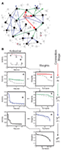Figure 6. Neuron-specific inputs robustly yield stable synaptic enhancement along several connection stages. (A) Schematic of post-synaptic connectivity of selected neurons up to stage four. Three neurons labeled a, b, c (pink) receive external inputs, neurons i, j, k serve as controls. Connectivity is only shown for neuron a. Red arrows depict the first connection stage, green second, blue third, and black the fourth. In this scheme, each neuron has three post-synaptic connections. As these are randomly drawn, neurons at all stages may connect to each other. (B) Neural activities (left column) and synaptic weights (right column) found for the first stages of post-synaptic connectivity. Three inputs, to a, b, c, are compared to three controls i, j, and k. Same color code as in (A). Values are rank ordered as this provides a clearer picture than a histogram, which is distorted by the binning. Weights descending from input neurons are significantly different from those descending from the control neurons up to the third-connection stage (Kolmogorov-Smirnov test, p < 0.01, asterisks), activities are different up to the second stage. Total number of connections when starting with three neurons are 9, 27, 81, and 243 along the first four stages (right). Parameters: Network size N = 100, connectivity 3%, initial activation of controls on average u = 0.005 and of inputs u = 0.05, vT = 0.1, time scale of conventional plasticity relative to scaling μ/γ = 5, 20% global inhibition is performed by decreasing activity of each neuron according to 20% of the mean activity of the network.

For instance, if some specific neurons (cf. those labeled a, b, and c in Figure 6A) receive strong external input signals, while all others only receive weak random inputs, these specific neurons initiate a sequence of increased post-synaptic connections that emerge after a learning phase (Figure 6B). This is different for all other neurons (cf. neurons labeled i, j, k, which serve as controls). For those and their post-synaptic targets, connectivity remains low and random along several connection stages.

Stimulating neurons thus initiates an activity trace propagating from the stimulated neurons to their post-synaptic targets, which in turn transmit the activity to their own post-synaptic neurons and so on, across several stages of post-synaptic connectivity. In our example of a moderately large, purely random circuit, neural activity is significantly enhanced up to the third stage of connectivity, compared to the same stages descending from initially un-stimulated control neurons. By changing the average connectivity in the network the number of enhanced stages changes, too. If the connectivity is larger than in the here shown example the external stimulus need less stages to reach the whole network and, therefore, less stages are significantly different from the control cases.

As the details of overall circuit connectivity are strongly random, a propagating signal may appear more than once at the same neuron. This leads to some exceptions from reliable transmission, if – due to overlapping post-synaptic connectivity – some neurons in the post-synaptic stages of control neurons are also located in a post-synaptic stage of a stimulated neuron. In Figure 6, this effect is illustrated on purpose for the control neuron j, Figure 6A.

Taken together, these results demonstrate that combining conventional plasticity with non-linearly weight-dependent synaptic scaling enables a neural circuit to faithfully represent a specific input pattern, across several stages even if the wiring is unstructured and randomly.

This may appear remarkable because at later post-synaptic stages already a large fraction of the entire network may be active (79 out of 100 neurons at the third post-synaptic stage in our example). Thus, the information contained in the inputs has spread over almost the entire circuit. In spite of this, synapses may still show a statistically significant trace of the input (Kolmogorov-Smirnov test, asterisk, p < 0.01). Such specific propagation robustly occurs across several orders of magnitude of the input and control activities and also systematically changes with varying vT. The same signal propagation behavior occurs also without inhibition, albeit requiring small values for vT (data not shown).

Table 2 shows mean values at the different connectivity stages averaged over ten numerical experiments. The Kolmogorov-Smirnov test was used to assess whether input- and control-distributions are different, which is confirmed at p < 0.01 for all but the last stages (see asterisk markers in figure and table). This general picture is expected because at the forth stage the input activity has been dispersed over about 98 neurons. Dispersion depends on the relation between connectivity and network size. Tests for different networks confirm that – as expected – inputs are represented across more stages in large networks with low connectivity and vice versa (data not shown). Thus, synaptic scaling combined with conventional forms of plasticity enables random neural circuits to represent input patterns at specific neurons across several of their post-synaptic connection stages.

TABLE 2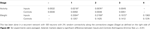Table 2. Statistical evaluation of the storage of an input representation from three inputs (Inputs) compared to three randomly chosen controls (Controls).

These results suggest using such networks also in behaving systems. The simultaneous stabilization of synapses and behavior is a very difficult problem as the behavior creates an ongoing non-steady state situation reflected at the inputs of the network. It is, however, possible to embed an initially randomly wired network in a sensori-motor loop and demonstrate that synapses self-organize into stable input-driven patterns and that the agent learns appropriate behavior.

4. Discussion

The tendency of real neural networks to achieve firing rate homeostasis by synaptic scaling (Turrigiano et al., 1998; Abbott and Nelson, 2000; Turrigiano and Nelson, 2000, 2004; Stellwagen and Malenka, 2006) has been discussed by these authors as a potential solution to the problem of regulating synaptic weight growth. The current study has shown that plasticity mechanisms augmented by an additive scaling term will lead to synaptic stability but maintain synaptic diversity. Conventionally, plasticity rules are extended by stabilization terms (for example subtractive or multiplicative terms) to assure limited weight growth (Sejnowski and Tesauro, 1989; Miller and MacKay, 1994; Gerstner and Kistler, 2002). Different biophysical mechanisms exist, which achieve this (Desai et al., 1999). In this article we identified the broad and general stabilizing properties of synaptic scaling. Scaling can stabilize a network either independently or in conjunction with other weight regularization mechanisms.

All conventional, correlation-based plasticity rules without additional stabilization terms (e.g., Hebb, Anti-Hebb, STDP, BCM, and others) are limited to a second order weight dependence (Gw2). Thus, the second order form G = aw2 + bw characterizes all generic plasticity mechanisms considered in this work. Other plasticity rules have been formulated to explicitly include weight stabilization terms [for example Oja’s rule (Oja, 1982), or rules with subtractive weight normalization (Miller and MacKay, 1994)]. Those rules by construction contain higher order terms that may be stabilizing, even without synaptic scaling. At this point the literature provides a diverse and non-congruent picture about the mathematical form of such terms. In general, one finds that stable fixed points arise for all “correctly constructed” plasticity rules which contain a third order weight dependency. There, the third order needs to be introduced a compensatory, convergent (e.g., negative, divisive) term. Some more recent approaches [e.g., Clopath et al. (2010)] successfully make use of this fixed point behavior implicitly.

The situation becomes even more diverse when considering STDP. The weight stabilization properties of this mechanism have been discussed in many studies and different additional mechanisms have been suggested to improve on this intrinsic property [like weight dependent STDP (Van Rossum et al., 2000) or hard boundaries (Song et al., 2000)]. The current study does not produce any conflict with these results as scaling can co-act with any plain or extended STDP rule. Whether or not such an interaction indeed exists could be measured as suggested in Figure 3. In particular, the strong link between STDP and BCM as discussed next should be considered here. The long time-scale of scaling might indeed remove the distinction between STDP and BCM rules.

Izhikevich and Desai showed in 2003 that BCM and STDP are strongly related (Izhikevich and Desai, 2003). This can be understood if we consider the mean firing rate v of the post-synaptic neuron over a long time interval and distribute the spikes equidistantly in time. This leads to a situation where a high post-synaptic firing rate results in LTP (v > Θ) and a low rate in LTD (v < Θ). This relation holds only for nearest-neighbor STDP (spike-to-spike association), but Pfister and Gerstner (2006) showed that STDP with triplets (three spikes leading to a weight change) is under some conditions equal to BCM, too. In the context of this study one must consider that synaptic scaling is a rather slow process. Thus, immediate effects of STDP, which happen on a much shorter time scale are expected to average out. As a consequence, we expect that on the time scale of synaptic scaling an existing STDP characteristic may indeed be represented by a BCM rule.

Conventionally the BCM rule is endowed with a sliding threshold Θ (Bienenstock et al., 1982). By this the rule is stabilized. We have omitted this aspect and investigate the constant-threshold BCM rule instead. The reason for this is that such a sliding Θ corresponds to a shift of the LTP and/or LTD windows along the ΔT axis in STDP. For wSTDP [weight-dependent STDP, Van Rossum et al. (2000)] the problem is comparable to the sliding threshold scheme. Here the LTD part is weight dependent, resulting in stronger depression for larger synapses. Therefore, again the LTP and LTD windows are changed over time. There is however no clear-cut experimental evidence existing for temporal shifts of the STDP window over long time scales. Thus, the constant-threshold BCM rule may well represent the most generic STDP-compatible rule when considering scaling mechanisms.

Our study indicates that synaptic scaling with sufficiently strong, convex non-linear weight dependence yields stabilization in the presence of all forms of conventional plasticity. This offers a unifying alternative to the currently existing manifold of theories of how synaptic plasticity may be stabilized in neural systems.

Earlier studies have suspected a general dependence of scaling on the current synaptic weight w itself (Abbott and Nelson, 2000; Turrigiano and Nelson, 2004). Our analysis suggests that synaptic scaling actually needs to be weight-dependent and must increase with weight to ensure stability and diversity, a prediction that may be tested experimentally. For plain Hebbian plasticity, positive synapses globally stabilize if scaling is linearly weight dependent (Figure 1B). Generic stabilization also for other plasticity mechanisms is observed for non-linear convex scaling (Figures 1C,D). Little is known to what degree Hebbian mechanisms would also apply to inhibitory synapses (Kullmann and Lamsa, 2007). The analysis also shows that the BCM rule together with synaptic scaling excludes inhibitory synapses as they tend to converge to zero. Thus, stabilization of Hebbian plasticity by a linear synaptic scaling mechanism may indeed represent the most prevalent constellation in real networks. The dependency on w makes scaling a synapse-dependent mechanism as suggested earlier (Rabinowitch and Segev, 2006, 2008). Future experimental studies could address this question by measuring the exponent in Hwn. Currently there are several mechanisms discussed how synaptic scaling might be biophysically realized in neural systems, most of which favor changes in AMPA receptor accumulation at the synaptic site (Turrigiano and Nelson, 1998, 2004). It remains, thus, an interesting question whether and how such an accumulation process would depend on the actual status of the synaptic weight.

Another experimentally measurable parameter is the target firing rate vT. As mentioned in the “Materials and Methods Section,” the mean firing rate of a neuron will normally not be equal to vT as synaptic plasticity shifts the synaptic weights and, therefore, the activity to higher values. Thus, only by switching off all synaptic plasticity without influencing the biological mechanisms of synaptic scaling would lead to activities equal to the target firing rate of the synaptic scaling term.

For small groups of neurons (e.g., two or three cells), embedded in the large cortical network, some local connectivity patterns (called “synaptic motifs”) are over-represented as compared to their expected occurrence frequency (Sporns and Kötter, 2004; Bullmore and Sporns, 2009). Our results suggest that plasticity combined with synaptic scaling may naturally stabilize motifs. For instance, in Figure 4 we show that the simplest, bi-directional motif is stabilized depending on the input activity that arrives at the synapses. We conjecture that the synaptic scaling may stabilize more complex motifs, too.

Synaptic patterns (e.g., motifs) are, thus, related to activity patterns (Timme, 2007). The representation of activity patterns and their storage by learning mechanisms is a long-standing problem in neural network theory. In early studies storage has been robustly achieved for static patterns in time-discrete systems [e.g., Hopfield network, Hopfield (1982)]. Such approaches for static pattern representation are still dominating because so far it has been exceedingly difficult to achieve stable representations of spatial-temporal patterns in time-continuous systems. This is problematic, because the dynamic behavioral stability of animals but also of artificial agents (Steingrube et al., 2010) fundamentally relies on the stability of activation patterns, which need to be acquired and stabilized by learning. With spike-timing dependent plasticity it is possible to learn and stabilize weights (Song et al., 2000; Van Rossum et al., 2000). As a consequence STDP has been discussed as a potential mechanism to store input patterns in a network in a stable way thereby creating cell assemblies (Izhikevich, 2006). These assemblies would then activate as soon as the respective input is present (Hebb, 1949; Harris, 2005; Izhikevich, 2006). While promising, only a few studies have actually achieved pattern representation in such STDP-regulated networks. These studies, however, make rather strong, sometimes biophysically questionable assumptions about network topology (Bienenstock, 1995; Hertz and Prügel-Bennett, 1996; Diesmann et al., 1999; Jun and Jin, 2007), and plasticity mechanisms (Sougné, 2001; Matsumoto and Okada, 2002). The analysis here suggests that this is due to intrinsically instable fixed points in systems that change their synaptic weights with STDP without additional mechanisms [see also Kunkel et al. (2010)].

The current study shows that such a storage process is easily achieved by combining plasticity and scaling (Liu and Buonomano, 2009; Rossi Pool and Mato, 2010; Savin et al., 2010). Here, we have specifically addressed the hard problem of a randomly connected network, where recurrent connections in conjunction with synaptic dynamics are often assumed to imply instability (Rossi Pool and Mato, 2010). Contrary to this expectation, we found that a representation trace of the input emerges along several connection stages due to synaptic scaling (Figure 6). This phenomenon is highly robust against variations of the only three existing parameters (μ, γ, and vT) suggesting that plasticity combined with scaling may solve the problem of pattern representation in dynamic networks.

In the numerical experiments shown, network size and connectivity has been kept small to limit input dispersion and reduce simulation times. Note that already with 1% (Holmgren et al., 2003) to 10% connectivity, similar to that suggested for cortical networks, input dispersion is bounded such that in a micro-circuit of about 106 neurons, a single input may distribute across the entire network in only 2 stages. This estimate clearly shows that the formation of long (multi-stage) input traces by plasticity requires additional, biophysically justifiable developmental constraints on the network topology.

Experimental and theoretical studies have indeed shown that real networks follow certain spatial and topological constraints, which influence the formation of activity patterns (Eguiluz et al., 2005; Bonifazi et al., 2009; Bullmore and Sporns, 2009). It has been discussed (Bullmore and Sporns, 2009) that more complex, non-random topological features can be achieved in a generic way by structural plasticity (Poirazi and Mel, 2001; Chklovskii et al., 2004; Holtmaat and Svoboda, 2009), by which axons and dendrites are anatomically and, thus, functionally rearranged, sometimes over very long time scales.

As shown above, combining plasticity and scaling mechanisms allows generating and storing certain basic assembly structures and creating simple synaptic motifs. Structural plasticity might provide the additionally required mechanisms for obtaining topologically correct, more complex networks that exhibit functionally relevant activity patterns across many more network stages (Tetzlaff et al., 2010). Thus, the dynamic interaction between plasticity, synaptic scaling, and structural plasticity will be a highly relevant topic for future investigations. This study indicates that the stable formation of diverse synaptic patterns may well be possible in this case. This can lead to systems in which synapses and behavioral patterns are stabilized at the same time.

Conflict of Interest Statement

The authors declare that the research was conducted in the absence of any commercial or financial relationships that could be construed as a potential conflict of interest.

Acknowledgments

The research leading to these results has received funding from the European Community’s Seventh Framework Programme FP7/2007-2013 (Specific Programme Cooperation, Theme 3, Information and Communication Technologies) under grant agreement no. 270273, Xperience (Florentin Wörgötter), by the Federal Ministry of Education and Research (BMBF) by grants to the Bernstein Center for Computational Neuroscience (BCCN) – Göttingen, grant number 01GQ1005A, projects D1 and D2 (Florentin Wörgötter) and 01GQ1005B, project B3 (Marc Timme), by the Max Planck Research School for Physics of Biological and Complex Systems (Christian Tetzlaff). Authors are greatly thankful to Drs Minija Tamosiunaite and Tomas Kulvicius for fruitful feedback on this work.

References

Abbott, L. F., and Nelson, S. B. (2000). Synaptic plasticity: taming the beast. Nat. Neurosci. (Suppl.) 3, 1178–1183.

CrossRef Full Text

Abraham, W. C., and Bear, M. F. (1996). Metaplasticity: the plasticity of synaptic plasticity. Trends Neurosci. 19, 126–130.

Bender, V. A., and Feldman, D. E. (2006). A dynamic spatial gradient of Hebbian learning in dendrites. Neuron 51, 153–155.

Bi, G. Q., and Poo, M. M. (1998). Synaptic modifications in cultured hippocampal neurons: dependence on spike timing, synaptic strength, and postsynaptic cell type. J. Neurosci. 18, 10464–10472.

Bienenstock, E. L. (1995). A model of neocortex. Netw. Comput. Neural Syst. 6, 179–224.

CrossRef Full Text

Bienenstock, E. L., Cooper, L. N., and Munro, P. W. (1982). Theory for the development of neuron selectivity: orientation specificity and binocular interaction in visual cortex. J. Neurosci. 2, 32–48.

Bliss, T., and Lomo, T. (1973). Long-lasting potentiation of synaptic transmission in the dentate area of the anaesthetized rabbit following stimulation of the perforant path. J. Physiol. 232, 331–356.

Bonifazi, P., Goldin, M., Picardo, M. A., Jorquera, I., Cattani, A., Bianconi, G., Represa, A., Ben-Ari, Y., and Cossart, R. (2009). GABAergic hub neurons orchestrate synchrony in developing hippocampal networks. Science 326, 1419–1424.

Bullmore, E., and Sporns, O. (2009). Complex brain networks: graph theoretical analysis of structural and functional systems. Nat. Rev. Neurosci. 10, 186–198.

Chklovskii, D. B., Mel, B. W., and Svoboda, K. (2004). Cortical rewiring and information storage. Nature 431, 782–788.

Clopath, C., Büsing, L., Vasilaki, E., and Gerstner, W. (2010). Connectivity reflects coding: a model of voltage-based STDP with homeostasis. Nat. Neurosci. 13, 344–352.

Desai, N. S., Rutherford, L. C., and Turrigiano, G. G. (1999). Plasticity in the intrinsic excitability of cortical pyramidal neurons. Nature 2, 515–520.

Diesmann, M., Gewaltig, M.-O., and Aertsen, A. (1999). Stable propagation of synchronous spiking in cortical neural networks. Nature 402, 529–533.

Dudek, S., and Bear, M. (1993). Bidirectional long-term modification of synaptic effectiveness in the adult and immature hippocampus. J. Neurosci. 13, 2910–2918.

Eguiluz, V. M., Chialvo, D. R., Ceechi, G. A., Baliki, M., and Apkarian, A. V. (2005). Scale-free brain functional networks. Phys. Rev. Lett. 94, 018102.

Frömke, R. C., Poo, M.-M., and Dan, Y. (2005). Spike-timing-dependent synaptic plasticity depends on dendritic location. Nature 434, 221–225.

Gerstner, W., Kempter, R., van Hemmen, J. L., and Wagner, H. (1996). A neuronal learning rule for sub-millisecond temporal coding. Nature 383, 76–78.

Gerstner, W., and Kistler, W. M. (2002). Spiking Neuron Models: Single Neurons, Populations, Plasticity, Cambridge: Cambridge University Press.

Gütig, R., Aharonov, R., Rotter, S., and Sompolinsky, H. (2003). Learning input correlations through nonlinear temporally asymmetric hebbian plasticity. J. Neurosci. 23, 3697–3714.

Harris, K. D. (2005). Neural signatures of cell assembly organization. Nat. Rev. Neurosci. 6, 399–407.

Hebb, D. O. (1949). The Organization of Behaviour, New York: Wiley.

Hertz, J., and Prügel-Bennett, A. (1996). Learning synfire chains: turning noise into signal. Int. J. Neural Syst. 7, 445–450.

Holmgren, C., Harkany, T., Sevennenfors, B., and Zilberter, Y. (2003). Pyramidal cell communication within local networks in layer 2/3 of rat neocortex. J. Physiol. 551, 139–153.

Holtmaat, A., and Svoboda, K. (2009). Experience-dependent structural synaptic plasticity in the mammalian brain. Nat. Rev. Neurosci. 10, 647–658.

Hopfield, J. J. (1982). Neural networks and physical systems with emergent collective computational abilities. Proc. Natl. Acad. Sci. U.S.A. 79, 2554–2558.

Izhikevich, E. M. (2003). Simple model of spiking neurons. IEEE Trans. Neural Netw. 14, 1569–1572.

Izhikevich, E. M. (2004). Which model to use for cortical spiking neurons? IEEE Trans. Neural Netw. 15, 1063–1070.

CrossRef Full Text

Izhikevich, E. M. (2006). Polychronization: computation with spikes. Neural Comput. 18, 245–282.

Izhikevich, E. M., and Desai, N. S. (2003). Relating STDP to BCM. Neural Comput. 15, 1511–1523.

Jun, J. K., and Jin, D. Z. (2007). Development of neural circuitry for precise temporal sequences through spontaneous activity, axon remodeling, and synaptic plasticity. PLoS ONE 2, e723. doi: 10.1371/journal.pone.0000723

CrossRef Full Text

Kullmann, D. M., and Lamsa, K. P. (2007). Long term synaptic plasticity in hippocampal interneurons. Nat. Rev. Neurosci. 8, 687–699.

Kunkel, S., Diesmann, M., and Morrison, A. (2010). Limits to the development of feed-forward structures in large recurrent neuronal networks. Front. Comput. Neurosci. 4:160. doi: 10.3389/fncom.2010.00160

CrossRef Full Text

Liu, J., and Buonomano, D. (2009). Embedding multiple trajectories in simulated recurrent neural networks in self-organizing manner. J. Neurosci. 29, 13172–13181.

Magee, J. C., and Johnston, D. (1997). A synaptically controlled, associative signal for Hebbian plasticity in hippocampal neurons. Science 275, 209–213.

Markram, H., Lübke, J., Frotscher, M., and Sakmann, B. (1997). Regulation of synaptic efficacy by coincidence of postsynaptic APs and EPSPs. Science 275, 213–215.

Matsumoto, N., and Okada, M. (2002). Self-regulation mechanism of temporally asymmetric Hebbian plasticity. Neural Comput. 14, 2883–2902.

Miller, K. D., and MacKay, D. J. C. (1994). The role of constraints in Hebbian learning. Neural Comput. 6, 100–126.

CrossRef Full Text

Oja, E. (1982). A simplified neuron model as a principal component analyzer. J. Math. Biol. 15, 267–273.

Pfister, J.-P., and Gerstner, W. (2006). Triplets of spikes in a model of spike-timing-dependent plasticity. J. Neurosci. 26, 9673–9682.

Poirazi, P., and Mel, B. W. (2001). Impact of active dendrites and structural plasticity on the memory capacity of neural tissue. Neuron 29, 779–796.

Rabinowitch, I., and Segev, I. (2006). The endurance and selectivity of spatial patterns of long-term potentiation/depression in dendrites under homeostatic synaptic plasticity. J. Neurosci. 26, 13474–13484.

Rabinowitch, I., and Segev, I. (2008). Two opposing plasticity mechanisms pulling a single synapse. Trends Neurosci. 31, 377–383.

Rossi Pool, R., and Mato, G. (2010). Hebbian plasticity and homeostasis in a model of hypercolumn of the visual cortex. Neural Comput. 22, 1837–1859.

Savin, C., Joshi, P., and Triesch, J. (2010). Independent component analysis in spiking neurons. PLoS Comput. Biol. 6, e1000757. doi: 10.1371/journal.pcbi.1000757

CrossRef Full Text

Sejnowski, T. J., and Tesauro, G. (1989). Neural Models of Plasticity, Chapter The Hebb Rule for Synaptic Plasticity: Algorithms and Implementations, New York, NY: Academic Press, 94–103.

Sjöström, P., Turrigiano, G., and Nelson, S. (2001). Rate, timing, and cooperativity jointly determine cortical synaptic plasticity. Neuron 32, 1149–1164.

Sjöström, P. J., and Häusser, M. (2006). A cooperative switch determines the sign of synaptic plasticity in distal dendrites of neocortical pyramidal neurons. Neuron 51, 227–238.

Song, S., Miller, K. D., and Abbott, L. F. (2000). Competitive Hebbian learning through spike-timing-dependent synaptic plasticity. Nat. Neurosci. 3, 919–926.

Song, S., Sjöström, P. J., Reigl, M., Nelson, S. B., and Chklovskii, D. B. (2005). Highly nonrandom features of synaptic connectivity in local cortical circuits. PLoS Biol. 3, e68. doi: 10.1371/journal.pbio.0030068

CrossRef Full Text

Sougné, J. P. (2001). Connectionist Models of Learning, Development and Evolution, Chapter A Learning Algorithm for Synfire Chains, Heidelberg: Springer Verlag, 23–32.

Sporns, O., and Kötter, R. (2004). Motifs in brain networks. PLoS Biol. 2, e369. doi: 10.1371/journal.pbio.0020369

CrossRef Full Text

Steingrube, S., Timme, M., Wörgötter, F., and Manoonpong, P. (2010). Self-organized adaptation of a simple neural circuit enables complex robot behaviour. Nat. Phys. 6, 224–230.

CrossRef Full Text

Stellwagen, D., and Malenka, R. C. (2006). Synaptic scaling mediated by glial TNF-α. Nature 440, 1054–1059.

Tetzlaff, C., Okujeni, S., Egert, U., Wörgötter, F., and Butz, M. (2010). Self-organized criticality in developing neuronal networks. PLoS Comput. Biol. 6, e1001013. doi: 10.1371/journal.pcbi.1001013

CrossRef Full Text

Timme, M. (2007). Revealing network connectivity from response dynamics. Phys. Rev. Lett. 98, 224101.

Triesch, J. (2007). Synergies between intrinsic and synaptic plasticity mechanisms. Neural Comput. 19, 885–909.

Turrigiano, G. G., Leslie, K. R., Desai, N. S., Rutherford, L. C., and Nelson, S. B. (1998). Activity-dependent scaling of quantal amplitude in neocortical neurons. Nature 391, 892–896.

Turrigiano, G. G., and Nelson, S. B. (1998). Thinking globally, acting locally: AMPA receptor turnover and synaptic strength. Neuron 21, 933–935.

Turrigiano, G. G., and Nelson, S. B. (2000). Hebb and homeostasis in neuronal plasticity. Curr. Opin. Neurobiol. 10, 258–364.

CrossRef Full Text

Turrigiano, G. G., and Nelson, S. B. (2004). Homeostatic plasticity in the developing nervous system. Nat. Rev. Neurosci. 5, 97–107.

Van Rossum, M. C. W., Bi, G. Q., and Turrigiano, G. G. (2000). Stable Hebbian learning from spike-timing dependent plasticity. J. Neurosci. 20, 8812–8821.

Appendix

Used Neuron Models

In the following the equations and parameters of the different neuronal models used in the main text are presented. Fi activity of neuron i, wij weight connecting neuron i with j, Ii external input to neuron i.

Linear neuron model: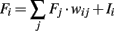Integrate and Fire model: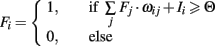Izhikevich “RS” neuron model: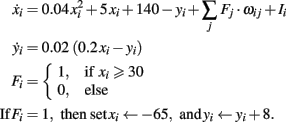Specific Fixed Point Calculations

With the definitions from the “Materials and Methods Section” it is possible to explicitly calculate fixed points for the specific cases. Thus, we show the final form of the fixed points for n = 2 for one synapse (Figure 1C), plain Hebbian plasticity (i.e., a = 0 and b = u2) and the simple neuronal activation functionThe conjoint plasticity and scaling mechanisms considered throughout the main text, then takes following form: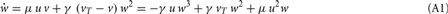and the fixed points are (for plots see Figure 1C):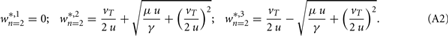Standard linear stability analysis (calculations not shown) demonstrates that w*,1 is unstable, w*,2 is stable, and w*,3 is stable if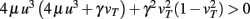; the latter generally holds because synaptic scaling acts substantially slower than conventional plasticity such that μ ≫ γ.

In the following, this analysis is expanded to a two synaptic system with plain Hebbian plasticity and a one and a two synaptic system with BCM.

Hebb-2: Two Synapses, Feed-Forward System with Plain Hebbian Plasticity

An example is shown in Figure 1D. The dynamical equations are given by: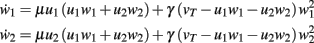Fixed points are calculated as: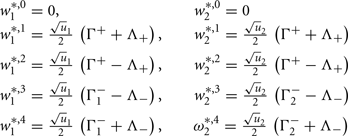with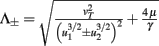,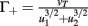,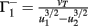, and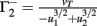. If u1 = u2 the denominators of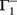and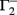become zero and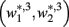and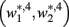do not exist.

BCM-1: One Synapse, Feed-Forward System with BCM Plasticity

Note, for brevity we do not calculate here the rather simple fixed points for n = 0 or n = 1 for the BCM rule. For n = 1 we only show the resulting plot in Figure 1E. The results of the following computations for n = 2 are illustrated in Figure 1F. The main equation is given by: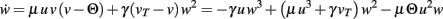Fixed points are calculated as: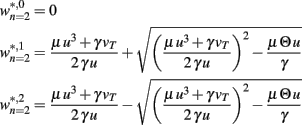BCM-2: Two Synapses, Feed-Forward System with BCM Plasticity

The main equations are given by: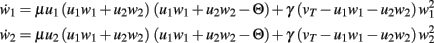Fixed points are calculated as: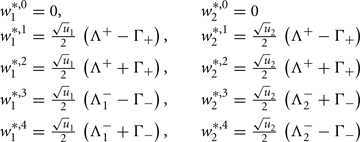with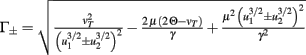and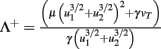,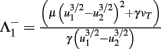and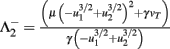.

Keywords: plasticity, neural network, homeostasis, synapse

Citation: Tetzlaff C, Kolodziejski C, Timme M and Wörgötter F (2011) Synaptic scaling in combination with many generic plasticity mechanisms stabilizes circuit connectivity. Front. Comput. Neurosci. 5:47. doi: 10.3389/fncom.2011.00047

Received: 02 August 2011; Accepted: 20 October 2011;
Published online: 10 November 2011.

Edited by:

David Hansel, University of Paris, France

Reviewed by:

Germán Mato, Centro Atomico Bariloche, Argentina
Sandro Romani, Columbia University, USA

Copyright: © 2011 Tetzlaff, Kolodziejski, Timme and Wörgötter. This is an open-access article subject to a non-exclusive license between the authors and Frontiers Media SA, which permits use, distribution and reproduction in other forums, provided the original authors and source are credited and other Frontiers conditions are complied with.

*Correspondence: Christian Tetzlaff, Network Dynamics Groups, Max Planck Institute for Dynamics and Self-Organization, Bunsenstr. 10, 37073 Göttingen, Germany. e-mail: tetzlaff@physik3.gwdg.de